Share

# Important Questions for BE Construction Engineering Semester 1 (FE First Year) - University of Mumbai - Engineering Mechanics

Subjects
Topics
Subjects
Popular subjects
Topics
Engineering Mechanics
< prev 1 to 20 of 84 next >

state and prove varigon’s theorem.

Appears in 1 question paper
Chapter:  System of Coplanar Forces
Concept: Varignon’s Theorem

Find the resultant of the force system as shown in the given figure.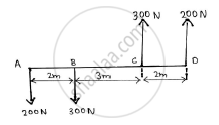Appears in 1 question paper
Chapter:  System of Coplanar Forces
Concept: Resultant of concurrent forces

Find the resultant of the force acting on the bell crank lever shown. Also locate its position with respect to hinge B.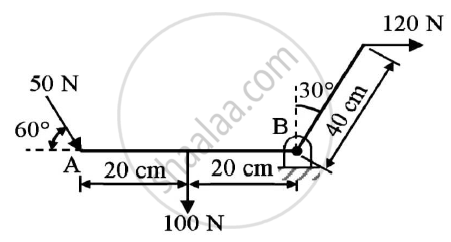Given : Forces on the bell crank lever
To find : Resultant and it’s position w.r.t hinge B

Appears in 1 question paper
Chapter:  System of Coplanar Forces
Concept: Resultant of parallel forces

In the rocket arm shown in the figure the moment of ‘F’ about ‘O’ balances that P=250 N.Find F.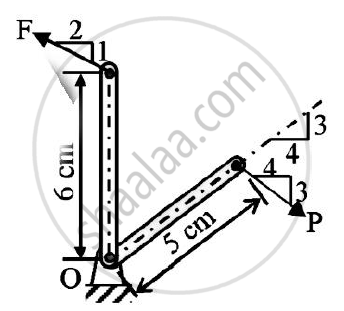Appears in 1 question paper
Chapter:  System of Coplanar Forces
Concept: Resultant of concurrent forces

Find the force F4 , so as to give the resultant of the force as shown in the figure given below.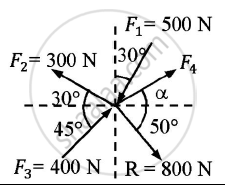Given : Forces and their resultant
To find : Force F4

Appears in 1 question paper
Chapter:  System of Coplanar Forces
Concept: Resultant of concurrent forces

The resultant of the three concurrent space forces at A is 𝑹̅ = (-788𝒋̅) N. Find the magnitude of F1,F2 and F3 forces.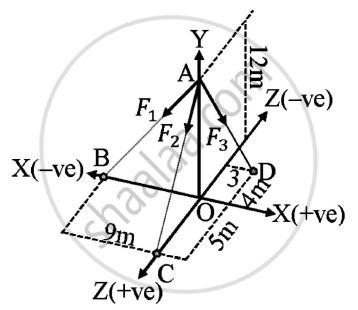Given : A=(0,12,0)
B=(-9,0,0)
C=(0,0,5)
D=(3,0,-4)
Resultant of forces = (-788𝑗̅) N
To find : Magnitude of forces F1,F2,F3

Appears in 1 question paper
Chapter:  System of Coplanar Forces
Concept: Resultant of concurrent forces

A force of 140 kN passes through point C (-6,2,2) and goes to point B (6,6,8). Calculate moment of force about origin.

Appears in 1 question paper
Chapter:  System of Coplanar Forces
Concept: Moment of force about a point

A machine part is subjected to forces as shown.Find the resultant of forces in magnitude and in direction.
Also locate the point where resultant cuts the centre line of bar AB.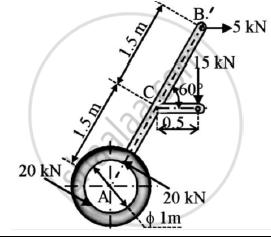Solution:
Given : A machine subjected to various forces
To find : Resultant of forces
Point where the resultant force cuts the bar AB

Appears in 1 question paper
Chapter:  System of Coplanar Forces
Concept: Varignon’s Theorem

Find the resultant of the parallel force system shown in Figure 1 and locate the same with respect to point C.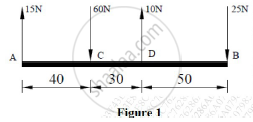Appears in 1 question paper
Chapter:  System of Coplanar Forces
Concept: Resultant of parallel forces

A force of magnitude of 20kN, acts at point A(3,4,5)m and has its line of action passing through B(5,-3,4)m. Calculate the moment of this force about a line passing through points S(2,-5,3) m and T(-3,4,6)m.

Appears in 1 question paper
Chapter:  System of Coplanar Forces
Concept: Moment of force about a point

Determine the position of the centroid of the plane lamina. Shaded portion is removed.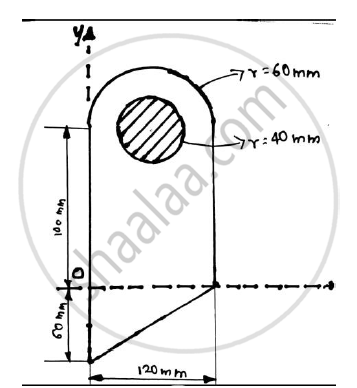Appears in 1 question paper
Chapter:  Center of Gravity and Centroid for Plane Laminas
Concept: Centroid for Plane Laminas

Find the centroid of the shaded portion of the plate shown in the figure.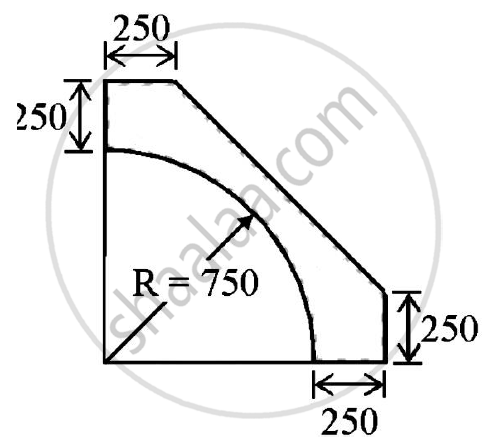Appears in 1 question paper
Chapter:  Center of Gravity and Centroid for Plane Laminas
Concept: Centroid for Plane Laminas

For the composite lamina shown in the figure, determine the coordinates of its centroid.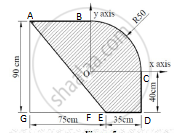Appears in 1 question paper
Chapter:  Center of Gravity and Centroid for Plane Laminas
Concept: Centroid for Plane Laminas

Determine the reaction at points of constant 1,2 and 3. Assume smooth surfaces.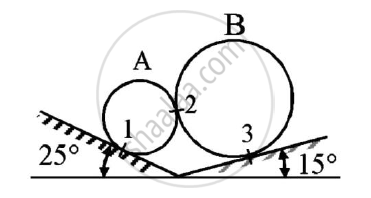Given: The spheres are in equilibrium
To find: Reactions at points 1,2 and 3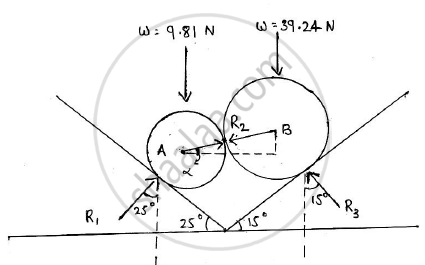Appears in 1 question paper
Chapter:  Equilibrium of System of Coplanar Forces
Concept: Condition of Equilibrium for non-concurrent nonparallel general forces

Explain the conditions for equilibrium of forces in space.

Appears in 1 question paper
Chapter:  Equilibrium of System of Coplanar Forces
Concept: Condition of equilibrium for concurrent forces

Find the support reactions at A and B for the beam loaded as shown in the given figure.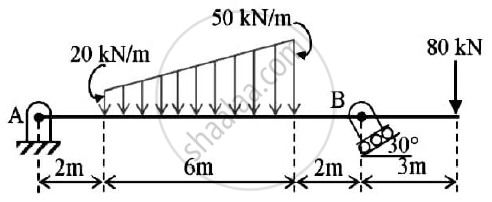Appears in 1 question paper
Chapter:  Equilibrium of System of Coplanar Forces
Concept: Condition of equilibrium for parallel forces

Determine the force P required to move the block A of 5000 N weight up the inclined plane, coefficient of friction between all contact surfaces is 0.25. Neglect the weight of the wedge and the wedge angle is 15 degrees.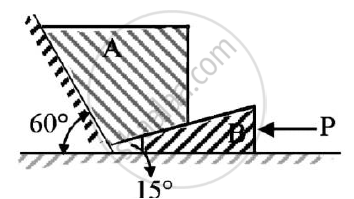Given : Weight of block A = 5000 N
μs=0.25
Wedge angle = 15º

To find : Force P required to move block A up the inclined plane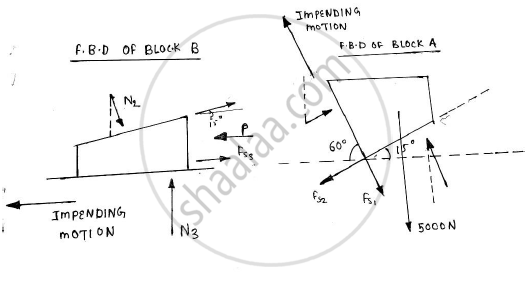Appears in 1 question paper
Chapter:  Equilibrium of System of Coplanar Forces
Concept: Condition of equilibrium for concurrent forces

State Lami’s theorem.
State the necessary condition for application of Lami’s theorem.

Appears in 1 question paper
Chapter:  Equilibrium of System of Coplanar Forces
Concept: Condition of equilibrium for parallel forces

Two spheres A and B of weight 1000N and 750N respectively are kept as shown in the figure..Determine reaction at all contact points 1,2,3 and 4. Radius of A is 400 mm and radius of B is 300 mm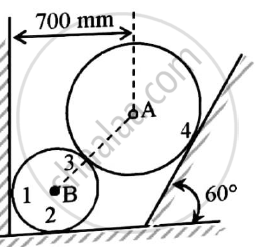Given  : Two spheres are in equilibrium
W1=1000 N
W2=750 N
rA=400 mm
rB=300 mm
To find : Reaction forces at contact points 1,2,3 and 4

Appears in 1 question paper
Chapter:  Equilibrium of System of Coplanar Forces
Concept: Condition of Equilibrium for non-concurrent nonparallel general forces

Refer to figure.If the co-efficient of friction is 0.60 for all contact surfaces and θ = 30o,what force P applied to the block B acting down and parallel to the incline will start motion and what will be the tension in the cord parallel to inclined plane attached to A.
Take WA=120 N and WB=200 N.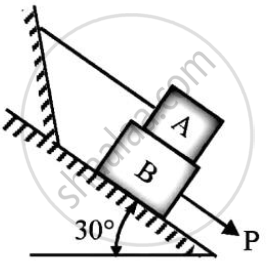Appears in 1 question paper
Chapter:  Equilibrium of System of Coplanar Forces
Concept: Condition of equilibrium for concurrent forces
< prev 1 to 20 of 84 next >
Important Questions for BE Construction Engineering Semester 1 (FE First Year) University of Mumbai Engineering Mechanics. You can further filter Important Questions by subjects and topics. Chapter wise important Questions for Semester 1 (FE First Year) University of Mumbai. it gets easy to find all Semester 1 (FE First Year) important questions with answers in a single place for students. Saving time and can then focus on their studies and practice. Important questions for Semester 1 (FE First Year) chapter wise with solutions

Semester 1 (FE First Year) Engineering Mechanics can be very challenging and complicated. But with the right ideas and support, you will be able to make it work. That’s why we have the semester 1 (fe first year) Engineering Mechanics important questions with answers pdf ready for you. All you need is to acquire the PDF with all the content and then start preparing for the exam. It really helps and it can bring in front an amazing experience every time if you're tackling it at the highest possible level.

## Engineering Mechanics exams made easy

We make sure that you have access to the important questions for Semester 1 (FE First Year) Engineering Mechanics B.E. University of Mumbai. This way you can be fully prepared for any specific question without a problem. There are a plethora of different questions that you need to prepare. And that's why we are covering the most important ones. They are the questions that will end up being more and more interesting and the results themselves can be staggering every time thanks to that. The quality itself will be quite amazing every time, and you can check the important questions for Semester 1 (FE First Year) Engineering Mechanics University of Mumbai whenever you see fit.

The important questions for Semester 1 (FE First Year) Engineering Mechanics 2020 we provide on this page are very accurate and to the point. You will have all the information already prepared and that will make it a lot simpler to ace the exam. Plus, you get to browse through these important questions for Semester 1 (FE First Year) Engineering Mechanics 2020 B.E. and really see what works, what you need to adapt or adjust and what still needs some adjustment in the long run. It's all a matter of figuring out these things and once you do that it will be a very good experience.

All you have to do is to browse the important questions for upcoming University of Mumbai Semester 1 (FE First Year) 2020 on our website. Once you do, you will know what needs to be handled, what approach works for you and what you need to do better. That will certainly be an incredible approach and the results themselves can be nothing short of staggering every time. We encourage you to browse all these amazing questions and solutions multiple times and master them. Once you do that the best way you can, you will have a much higher chance of passing the exam, and that's what really matters the most!

S